Home

# Division Algebras of Prime Degree and Maximal Galois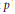$p$ -Extensions

## Abstract

Let$p$ be an odd prime number, and let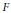$F$ be a field of characteristic not$p$ and not containing the group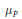${{\mu }_{p}}$ of$p$ -th roots of unity. We consider cyclic$p$ -algebras over$F$ by descent from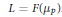$L\,=\,F\left( {{\mu }_{p}} \right)$ . We generalize a theorem of Albert by showing that if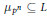${{\mu }_{{{p}^{n}}}}\,\subseteq \,L$ , then a division algebra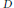$D$ of degree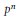${{p}^{n}}$ over$F$ is a cyclic algebra if and only if there is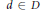$d\,\in \,D$ with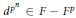${{d}^{{{P}^{n}}}}\,\in \,F\,-\,{{F}^{P}}$ . Let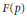$F(p)$ be the maximal$p$ -extension of$F$ . We show that$F(p)$ has a noncyclic algebra of degree$p$ if and only if a certain eigencomponent of the$p$ -torsion of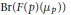$\text{Br(F(p)(}{{\mu }_{p}}\text{))}$ is nontrivial. To get a better understanding of$F(p)$ , we consider the valuations on$F(p)$ with residue characteristic not$p$ , and determine what residue fields and value groups can occur. Our results support the conjecture that the$p$ torsion in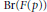$\text{Br}(F(p))$ is always trivial.

• # Send article to Kindle

Note you can select to send to either the @free.kindle.com or @kindle.com variations. ‘@free.kindle.com’ emails are free but can only be sent to your device when it is connected to wi-fi. ‘@kindle.com’ emails can be delivered even when you are not connected to wi-fi, but note that service fees apply.

Find out more about the Kindle Personal Document Service.

Division Algebras of Prime Degree and Maximal Galois$p$-Extensions Available formats × # Send article to Dropbox To send this article to your Dropbox account, please select one or more formats and confirm that you agree to abide by our usage policies. If this is the first time you use this feature, you will be asked to authorise Cambridge Core to connect with your <service> account. Find out more about sending content to Dropbox. Division Algebras of Prime Degree and Maximal Galois$p$ -Extensions
Available formats
×

# Send article to Google Drive

To send this article to your Google Drive account, please select one or more formats and confirm that you agree to abide by our usage policies. If this is the first time you use this feature, you will be asked to authorise Cambridge Core to connect with your <service> account. Find out more about sending content to Google Drive.

## Metrics

### Full text viewsFull text views reflects the number of PDF downloads, PDFs sent to Google Drive, Dropbox and Kindle and HTML full text views.

Total number of HTML views: 0
Total number of PDF views: 0 *Loading metrics...

### Abstract viewsAbstract views reflect the number of visits to the article landing page.

Total abstract views: 0 *Loading metrics...

* Views captured on Cambridge Core between <date>. This data will be updated every 24 hours.

Usage data cannot currently be displayed.